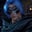Related Tags

pandas

# How to find the uncommon rows between two DataFrames in pandasGutha Vamsi Krishna

### Overview

A DataFrame is a two-dimensional data structure where data is aligned in rows and columns. In this answer, we'll learn to find the uncommon rows between the two data frames using pandas.

To find out uncommon rows, we follow the steps below:

• Merge the two DataFrames.
• Remove the duplicates from the resulted DataFrame in the above step.

We can merge two DataFrames using the concat() method and remove duplicates using the drop_duplicates() method.

### Syntax

concat([list of dataframes separated by commas])

drop_duplicates()

### Parameters

The concat() method takes a list of DataFrames as a parameter.

### Return value

It returns a new DataFrame appending all of them.

The drop_duplicates() method takes optional parameters like keep, which decides what value to keepfirst, last, False while removing the duplicates and returns a DataFrames without duplicates.

### Example

import pandas as pd

#data frame 1
classA = pd.DataFrame(
{
"Student": ['John', 'Lexi', 'Augustin', 'Jane', 'Kate'],
"Age": [18, 17, 19, 17, 18]
}
)

#data frame 2
classB = pd.DataFrame(
{
"Student": ['John', 'Lexi', 'Bob', 'karl', 'Kate'],
"Age": [18, 17, 16, 19, 18]
}
)

#get uncommon rows
print(pd.concat([classA,classB]).drop_duplicates(keep=False))

### Explanation

• Line 1: We import the pandas module, which contains methods to create DataFrames and modify them.
• Line 4–9: We create a DataFrame that represents class A, which contains Student and their Age.
• Line 13–18: We create DataFrame that represents class B, which contains Student and their Age.
• Line 21: We filter the uncommon rows from the above two DataFrames. We use the concat() method to do so. In this method, we input DataFrames in a list as a parameter to it and remove duplicate rows from the resultant data frame using the drop_duplicates() method.

RELATED TAGS

pandas

CONTRIBUTORGutha Vamsi Krishna